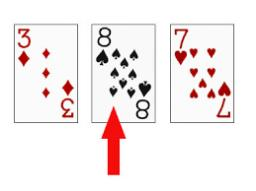# Multiply 2874

I think the number. If you multiply it by seven and add 3 to the product, you get 80. What number do I think?

x =  11

### Step-by-step explanation:

7x+3=80

7·x+3=80

7x = 77

x = 77/7 = 11

x = 11

Our simple equation calculator calculates it.Did you find an error or inaccuracy? Feel free to write us. Thank you!

Tips for related online calculators
Do you have a linear equation or system of equations and looking for its solution? Or do you have a quadratic equation?